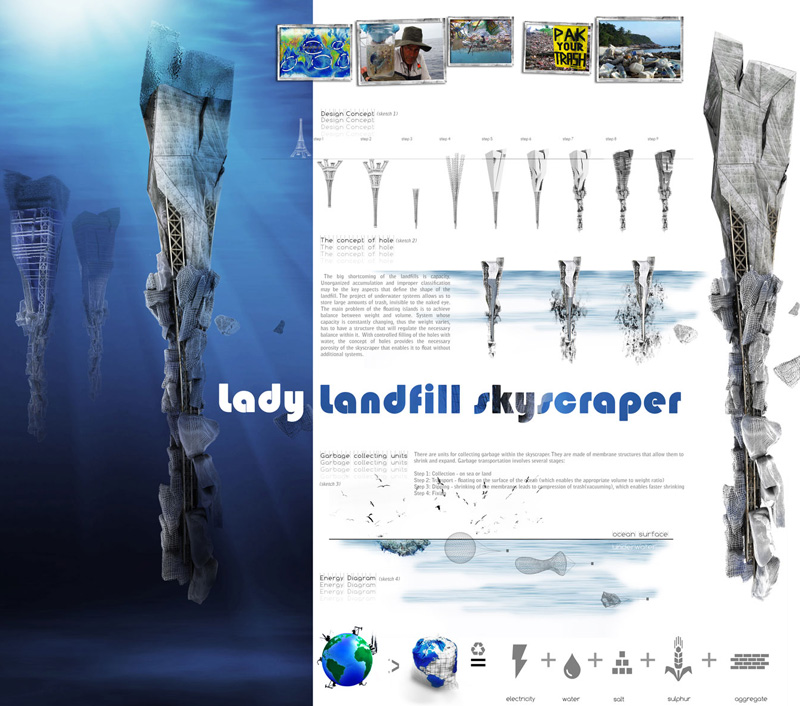Curve/Division/Divide LengthThe Divide Length tool is used to divide a curve into segments of a predetermined length. The C variable is the curve that you are wanting to divide and the L variable is the length of each division (determined by a slider). P exports the coordinates of each division point, which I used to draw a line to an arbitrary point that I placed.

p2_Brad Callahan 02 & Tim Shamblin 01